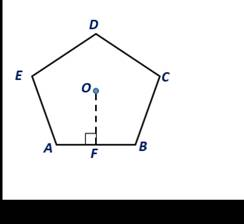Chapter 8.CT, Problem 9CTElementary Geometry For College St...

7th Edition
Alexander + 2 others
ISBN: 9781337614085

Solutions

Chapter
SectionElementary Geometry For College St...

7th Edition
Alexander + 2 others
ISBN: 9781337614085
Textbook Problem

A regular pentagon has an apothem of length 4.0 in. and each side is of length 5.8 in. For the regular pentagon, find its:a) Perimeterb) AreaTo determine

a.

To find:

The perimeter of the regular pentagon.

Explanation

Given:

A regular pentagon has an apothem of length 4.0 in. and each side is of length 5.8 in. For the regular pentagon.

Definition:

For a regular polygon of n sides, each side of length s, its perimeter is P=ns.

Calculation:

Given that the length of the side of the regular pentagon is 5

To determine

b.

To find:

The area of the regular pentagon.

Still sussing out bartleby?

Check out a sample textbook solution.

See a sample solution

The Solution to Your Study Problems

Bartleby provides explanations to thousands of textbook problems written by our experts, many with advanced degrees!

Get Started

Evaluate the integrals in Problems 1-32. 12.

Mathematical Applications for the Management, Life, and Social Sciences

Convert from degrees to radians. (a) 300 (b) 18

Single Variable Calculus: Early Transcendentals, Volume I

In Exercises 4143, find the distance between the two points. 42. (9, 6) and (6, 2)

Applied Calculus for the Managerial, Life, and Social Sciences: A Brief Approach

Evaluate the integral. 57. x3x+cdx

Single Variable Calculus: Early Transcendentals